• Posts tagged "pacf"

# Blog Archives

## R语言解读自回归模型

R的极客理想系列文章，涵盖了R的思想，使用，工具，创新等的一系列要点，以我个人的学习和体验去诠释R的强大。

R语言作为统计学一门语言，一直在小众领域闪耀着光芒。直到大数据的爆发，R语言变成了一门炙手可热的数据分析的利器。随着越来越多的工程背景的人的加入，R语言的社区在迅速扩大成长。现在已不仅仅是统计领域，教育，银行，电商，互联网….都在使用R语言。

• 张丹(Conan), 程序员R,Nodejs,Java
• weibo：@Conan_Z
• blog: http://blog.fens.me
• email: bsspirit@gmail.com

http://blog.fens.me/r-ar1. 自回归模型介绍
2. 用R语言构建自回归模型
3. 模型识别ACF/PACF
4. 模型预测

## 1. 自回归模型(AR)

p阶自回归过程的公式定义：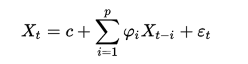• Xt是当期的X的表现
• c是常数项
• p是阶数，i为从1到p的值
• φi是自相关系数
• t为时间周期
• εt是均值为0，标准差为δ 的随机误差，同时δ是独立于t的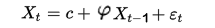• 必须具有平稳性，平稳性要求随机过程的随机特征不随时间变化。
• 必须具有自相关性，如果自相关系数(φi)小于0.5，则不宜采用，否则预测结果极不准确。
• 自回归只适用于预测与自身前期相关的现象，即受自身历史因素影响较大的现象。对于受其他因素影响的现象，不宜采用自回归，可以改用向量自回归模型。

• 随机变量Yt的均值和方差均与时间t无关
• 随机变量Yt和Ys的协方差只与时间差（步长）t-s有关
• 对于平稳时间序列在数学上有比较丰富的处理手段，非平稳的时间序列通过差分等手段转化为平稳时间序列处理

## 2. 用R语言构建自回归模型

``````
# 随机游走的数据集
> set.seed(0)
> x<-w<-rnorm(1000)       # 生成符合正态分布N(0,1)的数据
> for(t in 2:1000) x[t]<-x[t-1]+w[t]
> tsx<-ts(x)              # 生成ts时间序列的数据集

# 查看数据集
 1.2629543 0.9367209 2.2665202 3.5389495 3.9535909 2.4136409
 1.4850739 1.1903534 1.1845862 3.5892396 4.3528331 3.5538238
 2.4061668 2.1167053 1.8174901

> plot(tsx)            # 生成可视化图形
> a<-ar(tsx);a         # 进行自回归建模

Call:
ar(x = tsx)

Coefficients:
1
0.9879

Order selected 1  sigma^2 estimated as  1.168
``````

R语言中ar()函数提供了多种自相关系数的估计，包括"yule-walker", "burg", "ols", "mle", "yw"，默认是用yule-walker方法，常用的方法还有最小二乘法(ols)，极大似然法(mle)。

``````
> b<-ar(tsx,method = "ols");b

Call:
ar(x = tsx, method = "ols")

Coefficients:
1
0.9911

Intercept: -0.017 (0.03149)

Order selected 1  sigma^2 estimated as  0.9906
``````

``````
> d<-ar(tsx,method = "mle");d

Call:
ar(x = tsx, method = "mle")

Coefficients:
1
0.9904

Order selected 1  sigma^2 estimated as  0.9902
``````

## 3. 模型识别ACF/PACF

1. 自相关函数ACF(autocorrelation function)

ACF的公式为：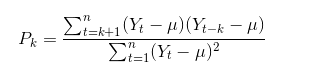• Pk,为ACF的标准误差
• t,为数据集的长度
• k,为滞后，取值从1到t-1，表示相距 k个时间间隔的序列值之间的相关性
• Yt,为样本在t时期的值
• Yt-k,为样本在t-k时期的值
• μ,为样本的均值

``````
> u<-mean(tsx)  #均值
> v<-var(tsx)   #方差

> # 1阶滞后
> p1<-sum((x[1:length(tsx)-1]-u)*(x[2:length(tsx)]-u))/((length(tsx)-1)*v);p1
 0.9878619
> # 2阶滞后
> p2<-sum((x[1:(length(tsx)-2)]-u)*(x[3:length(tsx)]-u))/((length(tsx)-1)*v);p2
 0.9760271
> # 3阶滞后
> p3<-sum((x[1:(length(tsx)-3)]-u)*(x[4:length(tsx)]-u))/((length(tsx)-1)*v);p3
 0.9635961
``````

``````
> acf(tsx)\$acf
, , 1

[,1]
[1,] 1.0000000
[2,] 0.9878619
[3,] 0.9760271
[4,] 0.9635961
[5,] 0.9503371
[6,] 0.9384022
[7,] 0.9263075
[8,] 0.9142540
[9,] 0.9024862
[10,] 0.8914740
[11,] 0.8809663
[12,] 0.8711005
[13,] 0.8628609
[14,] 0.8544984
[15,] 0.8462270
[16,] 0.8384758
[17,] 0.8301834
[18,] 0.8229206
[19,] 0.8161523
[20,] 0.8081941
[21,] 0.8009467
[22,] 0.7942255
[23,] 0.7886249
[24,] 0.7838154
[25,] 0.7789733
[26,] 0.7749697
[27,] 0.7709313
[28,] 0.7662547
[29,] 0.7623381
[30,] 0.7604101
[31,] 0.7577333
``````

``````
> acf(tsx)
``````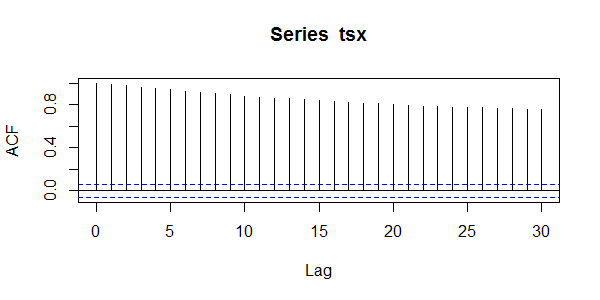2. 偏自相关函数(PACF)(partial autocorrelation function)

``````
> pacf(tsx)\$acf
, , 1

[,1]
[1,]  0.987861891
[2,]  0.006463542
[3,] -0.030541593
[4,] -0.041290415
[5,]  0.047921168
[6,] -0.009774246
[7,] -0.006267004
[8,]  0.002146693
[9,]  0.028782423
[10,]  0.014785187
[11,]  0.019307564
[12,]  0.060879259
[13,] -0.007254278
[14,] -0.004139848
[15,]  0.015707900
[16,] -0.018615370
[17,]  0.037067452
[18,]  0.019322565
[19,] -0.048471479
[20,]  0.023388065
[21,]  0.027640953
[22,]  0.051177900
[23,]  0.028063875
[24,] -0.003957142
[25,]  0.034030631
[26,]  0.004270416
[27,] -0.029613088
[28,]  0.033715973
[29,]  0.092337583
[30,] -0.031264028

# 可视化输出
> pacf(tsx)
``````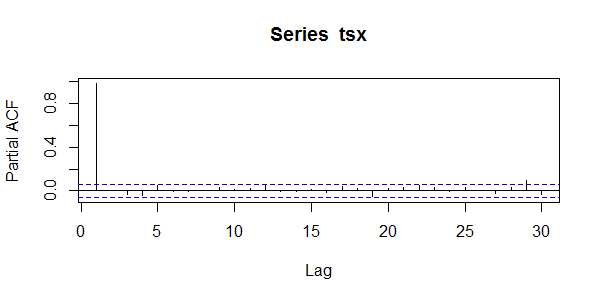## 4. 模型预测

``````
\$pred
Time Series:
Start = 2
End = 6
Frequency = 1
 9.839680 9.681307 9.524855 9.370303 9.217627

\$se
Time Series:
Start = 2
End = 6
Frequency = 1
 1.080826 1.519271 1.849506 2.122810 2.359189
``````

``````
# 生成50个预测值

# 把原数据画图
> plot(tsx)

# 把预测值和误差画出来
> lines(tsp\$pred,col='red')
> lines(tsp\$pred+tsp\$se,col='blue')
> lines(tsp\$pred-tsp\$se,col='blue')
``````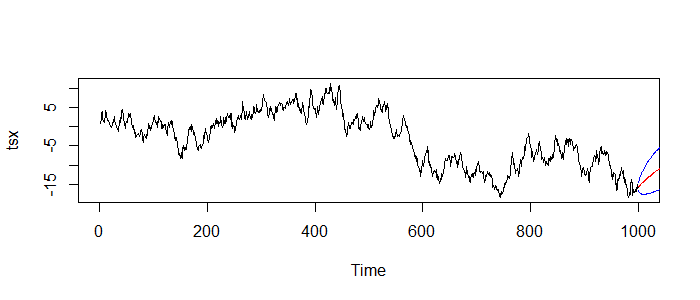``````
# 加载forecast包
> library('forecast')

# 生成模型AR(1)
> a2 <- arima(tsx, order=c(1,0,0))
> tsp2<-forecast(a2, h=50)
> plot(tsp2)
``````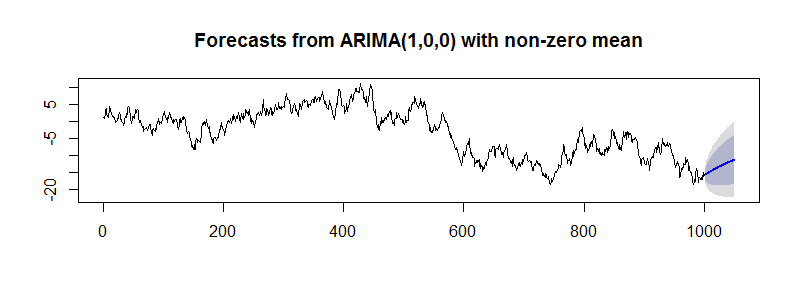``````
> tsp2
Point Forecast     Lo 80      Hi 80     Lo 95       Hi 95
1001      -15.71590 -16.99118 -14.440628 -17.66627 -13.7655369
1002      -15.60332 -17.39825 -13.808389 -18.34843 -12.8582092
1003      -15.49181 -17.67972 -13.303904 -18.83792 -12.1456966
1004      -15.38136 -17.89579 -12.866932 -19.22685 -11.5358726
1005      -15.27197 -18.06994 -12.474000 -19.55110 -10.9928432
1006      -15.16362 -18.21425 -12.112996 -19.82915 -10.4980922
1007      -15.05631 -18.33593 -11.776682 -20.07206 -10.0405541
1008      -14.95001 -18.43972 -11.460312 -20.28705  -9.6129750
1009      -14.84474 -18.52891 -11.160567 -20.47919  -9.2102846
1010      -14.74046 -18.60591 -10.875013 -20.65216  -8.8287673
1011      -14.63718 -18.67257 -10.601802 -20.80877  -8.4655994
1012      -14.53489 -18.73030 -10.339486 -20.95121  -8.1185723
1013      -14.43357 -18.78024 -10.086905 -21.08123  -7.7859174
1014      -14.33322 -18.82333  -9.843112 -21.20026  -7.4661903
1015      -14.23383 -18.86034  -9.607319 -21.30947  -7.1581923
1016      -14.13538 -18.89190  -9.378864 -21.40985  -6.8609139
``````

http://blog.fens.me/r-ar